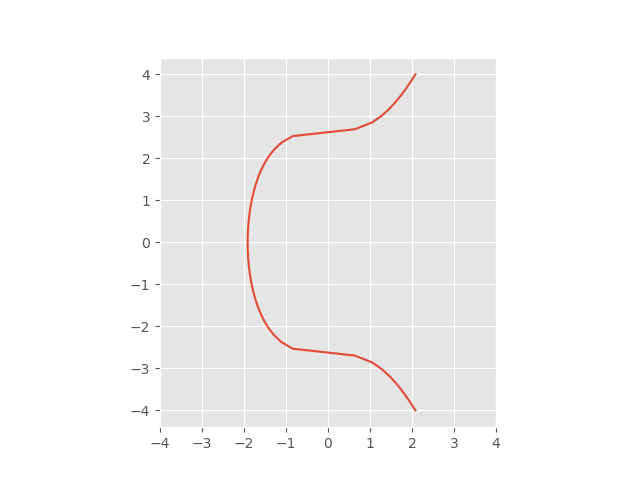# Bitcoin key mechanism and elliptic curves over finite fields

Bitcoin uses the Elliptic Curve Digital Signature Algorithm (ECDSA) based on elliptic curve cryptography. The particular elliptic curve is known as secp256k1, which is the curve

y² = x³ + 7

over a finite field (a.k.a. Galois field) to be described shortly.Addition on elliptic curves in the plane is defined geometrically in terms of where lines intercept the curve. We won’t go into the geometry here, except to say that it boils down to a set of equations involving real numbers. But we’re not working over real numbers; we’re working over a finite field.

## Finite field modulus

The idea is to take the equations motivated by the geometry in the plane then use those equations to define addition when you’re not working over real numbers but over a different field. In the case of secp256k1, the field is the finite field of integers mod p where

p = 2256 – 232 – 977

Here p was chosen to be relatively close to 2256. It’s not the largest prime less than 2256; there are a lot of primes between p and 2256. Other factors also went into the choice p. Note that we’re not working in the integers mod p per se; we’re working in an Abelian group whose addition law is defined by an elliptic curve over the integers mod p.

(Update: Here’s another post about secp256k1’s sister curve, secp256r1, another curve modulo a 256-bit prime, but with different structure.)

## Base point

Next, we pick a base point g on the elliptic curve. The standard defining secp256k1 says that g is

0279BE667EF9DCBBAC55A06295CE870B07029BFCDB2DCE28D959F2815B16F81798

in “compressed form” or

in “uncompressed form”.

The base point is a specially chosen point on the elliptic curve, and so it is a pair of numbers mod p, not a single number. How do you extract x and y from these compressed or uncompressed forms?

### Compressed form

The compressed form only gives x and you’re supposed to solve for y. The uncompressed form gives you x and y. However, the numbers are slightly encoded. In compressed form, the string either starts with “o2” or “o3” and the rest of the string is the hexadecimal representation of x. There will be two values of y satisfying

y² = x³ + 7 mod p

and the “o2” or “03” tells you which one to pick. If the compressed form starts with 02, pick the root whose least significant bit is even. And if the compressed form starts with 03, pick the root whose least significant bit is odd. (The two roots will add to p, and p is odd, so one of the roots will be even and one will be odd.)

### Uncompressed form

In either case we have

x = 79BE667EF9DCBBAC55A06295CE870B07029BFCDB2DCE28D959F2815B16F81798

and

We can verify this with a little Python code:

```    x = 0x79BE667EF9DCBBAC55A06295CE870B07029BFCDB2DCE28D959F2815B16F81798
p = 0xFFFFFFFFFFFFFFFFFFFFFFFFFFFFFFFFFFFFFFFFFFFFFFFFFFFFFFFEFFFFFC2F
assert((y*y - x*x*x - 7) % p == 0)
```

## Exponentiation over elliptic curve

Starting with our base point g, define kg to be g added to itself k times. Note again that the sense of “addition” here is addition in the elliptic curve, not addition in the field of integers mod p. The key to elliptic curve cryptography is that kg can be computed efficiently, but solving for k starting from the product kg cannot. You can compute kg using the fast exponentiation algorithm, but solving for k requires computing discrete logarithms. (This is the ECDLP: Elliptic Curve Discrete Logarithm Problem.)

Why is this called “exponentiation” and not “multiplication”? Arithmetic on the elliptic curve is commutative, and in commutative (i.e. Abelian) groups the group operation is usually denoted as addition. And repeated addition is called multiplication.

But in general group theory, the group operation is denoted as multiplication, and repeated application of the group operation is called  exponentiation. It’s conventional to use the general term “exponentiation” even though over an Abelian group it makes more sense to call it multiplication.

You undo exponentiation by taking logarithms, so the process of solving for k is called the discrete logarithm problem. The security of elliptic curve cryptography depends on the difficulty of computing discrete logarithms.

## Counting bits of security

The best algorithms for solving discrete logarithm problem in a group of size n currently require O(√n) operations. How big is n in our case?

The base point g was chosen to have a large order, and in fact its order is approximately 2256.  Specifically, the order of g written in hexadecimal is

n = FFFFFFFFFFFFFFFFFFFFFFFFFFFFFFFEBAAEDCE6AF48A03BBFD25E8CD0364141.

This means that we get approximately 256/2 = 128 bits of security because √(2256) = 2128.

## 4 thoughts on “Bitcoin key mechanism and elliptic curves over finite fields”

1. Hi John,

That was a really quick and good description of the concept on elliptic curves.

Maybe you could add a quick Definition of abelian groups or groups and fields in generel for people not that deep into group theory and algebra.

Best regards.

2. Lyian,

Thanks for the suggestion. I added links to pages on group theory and finite fields.

Jeremy,